# WBJEE Maths Test - 7

## 75 Questions MCQ Test WBJEE Sample Papers, Section Wise & Full Mock Tests | WBJEE Maths Test - 7

Description
Attempt WBJEE Maths Test - 7 | 75 questions in 120 minutes | Mock test for JEE preparation | Free important questions MCQ to study WBJEE Sample Papers, Section Wise & Full Mock Tests for JEE Exam | Download free PDF with solutions
QUESTION: 1

Solution:
QUESTION: 2

Solution:
QUESTION: 3

### The coefficient of x4 in the expansion of (1+x+x2+x3)11 is

Solution:
QUESTION: 4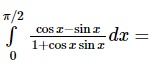Solution:
QUESTION: 5
The amplitude of sin(π/5) + i(1 - cos(π/5))is
Solution:
QUESTION: 6

The value of k for which the circles x2 + y2 -3x + ky - 5 = 0 and 4x2 + 4y2 - 12x - y - 9 = 0 becomes concentric is

Solution:
QUESTION: 7
If 2x-4y-9=0 and 6x-12y+7=0 are tangents to a circle, its radius is
Solution:
QUESTION: 8

(1 − i)6 + (1 − i)3 =

Solution:
QUESTION: 9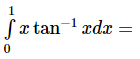Solution:
QUESTION: 10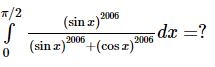Solution:
QUESTION: 11
If the system of equations x - ky - z = 0, kx - y - x = 0, x + y - z = 0 has a non-zero solution, then the possible values of k are
Solution:
QUESTION: 12

The solution of differential equation (dy/dx)=x2+sin3x is

Solution:
QUESTION: 13

If f x = A sin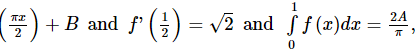then what is the value of B ?

Solution:
QUESTION: 14

The order and degree of the differential equation d2y/dx2 + (dy/dx)1/3 + x1=0 are respectively

Solution:
QUESTION: 15

If a + b + c = 0 and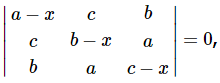then x =

Solution: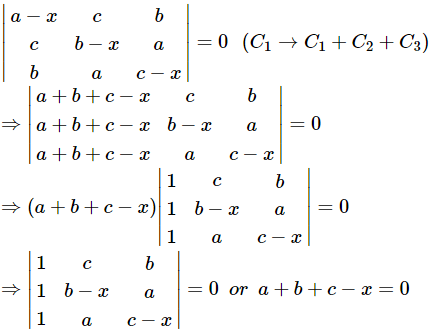⇒ x = a + b + c or 1(bc - bx - cx + x2 - a2) - c (c - x - a) + b (a - b + x) = 0
⇒ x = 0 or x2 + bc - bx - cx - a2 - c2 + cx + ac + ab - b2 + bx = 0
⇒ x = 0 or x2 = a2 + b2 + c2 - ab - bc - ca = a2 + b2 + c2 -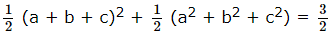(a2 + b2 c2)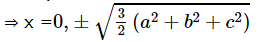QUESTION: 16
If f(x)=logx(logx), the value of f'(x) at x=c is
Solution:
QUESTION: 17

Pole of the line 2x + 3y + 4 = 0 w.r.t the ellipse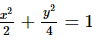is

Solution:
QUESTION: 18

If y=sin((1+x2)/(1-x2)), (dy/dx)=

Solution:
QUESTION: 19

The locus of the point of intersection of the perpendicular tangents to the ellipse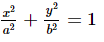is

Solution:
QUESTION: 20
The eccentricity of the hyperbola 9x2 - 16y2 + 72x - 32y - 16 = 0 is
Solution:
QUESTION: 21

The equation of the conic with focus at (1, -1), directrix along x - y + 1 = 0 and with eccentricity √2 is

Solution:
QUESTION: 22

Let f be a differentiable function such that,
f ′(x)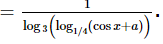If f(x) is increasing for all values of x then

Solution:
QUESTION: 23

What is the value of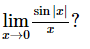Solution:
QUESTION: 24
The max value of the function sinx(1+cosx) is
Solution:
QUESTION: 25
For a square matrix A, it is given that AA' = I, then A is a
Solution:
QUESTION: 26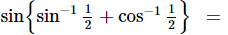Solution:
QUESTION: 27
If the normal to the parabola y2 = 4x at P(1, 2) meets the parabola again in Q, then Q =
Solution:
QUESTION: 28
Multiplication of a complex number z by i corresponds to (z ≠ o)
Solution:
QUESTION: 29

The focus of the parabola x2-8x+2y+7=0 is

Solution:
QUESTION: 30
A group of 7 is to be formed from 6 boys and 4 girls. In many ways can this be done if the boys are in majority?
Solution:
QUESTION: 31
The number of lines joining 8 points on a circle is
Solution:
QUESTION: 32

All letters of the word EAMCET can be written in all possible ways. In how many ways can the letters of the word EAMCET be arranged so that two vowels are never together.

Solution:

* M * C * T *
In places of *, E, A, E can be filled
No. of ways of filling M, C, T = 3! = 6
No. of ways of filling x by vowels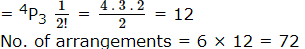QUESTION: 33
The chance of throwing a total of 7 or 12 with 2 dice, is
Solution:
QUESTION: 34

The probability of two events A and B are 0.25 and 0.40 respectively. The probability that both A and B occur is 0.15 . The probability that neither A nor B occurs is

Solution:
QUESTION: 35

The probability of getting a number between 1 and 100 which is divisible by one and itself only is

Solution:
QUESTION: 36

If to form a ΔABC it is given that a=5, b=7, sinA=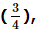, then possible triangles are

Solution: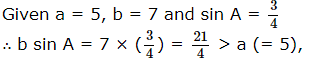Thus is this case no triangle in possible

QUESTION: 37

The maximum value of (x-p)2+(x-q)2+(x-r)2 will be at x is equal to

Solution:
QUESTION: 38

If a = 4, b = 3, angle A = 60º, then c is the root of the equation

Solution:
QUESTION: 39

If the product of the roots of the equation ax2+ 6x + α2 + 1 = 0 is -2 then α equals

Solution:
QUESTION: 40
The equation sin x + x cos x = 0 has at least one root in the interval
Solution: $\int \left(\mathrm{sin}x+x\mathrm{cos}x\right)dx=-\mathrm{cos}x+\int x\mathrm{cos}x\phantom{\rule{0.5em}{0ex}}dx=-\mathrm{cos}x+\left\{x\mathrm{sin}x-\int \mathrm{sin}x\phantom{\rule{0.5em}{0ex}}dx\right\}$
$=-\mathrm{cos}x+x\mathrm{sin}x+\mathrm{cos}x=x\mathrm{sin}x.$
$\text{Now},x\mathrm{sin}x=\text{0 has two roots}\phantom{\rule{0.5em}{0ex}}x=0,\phantom{\rule{0.5em}{0ex}}\pi .$
$\therefore \phantom{\rule{0.5em}{0ex}}\phantom{\rule{0.5em}{0ex}}\phantom{\rule{0.5em}{0ex}}\phantom{\rule{0.5em}{0ex}}\phantom{\rule{0.5em}{0ex}}$ its derived equation sin x + x cos x = 0 has a root lying between 0 and π
QUESTION: 41

The fourth, seventh and tenth terms of a G.P. are p, q and r respectively, then

Solution:
QUESTION: 42

If log 2,log (2x - 1),log (2x + 3) are in A.P.Then x is equal to

Solution:
QUESTION: 43
99th term of the series 2 + 7 + 14 + 23 + 34 ...is
Solution:
QUESTION: 44

Which of the following functions is not periodic?

Solution:
QUESTION: 45
Sum of infinite number of terms in G.P. is 20 and sum of their squares is 100. the common ratio of G.P is
Solution:
QUESTION: 46

The equation of perpendicular bisector of a line passing through the points (7,4) and (-1,-2) is

Solution: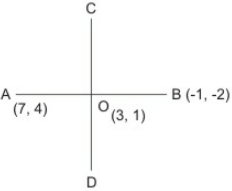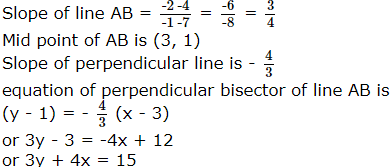QUESTION: 47

If f : R → R is given by f (x) = |x| and A = {x ∈ R : x < 0}, then f⁻1(A) equals

Solution:
QUESTION: 48
Angle between the lines y-2x=9 and x+2y=-7 is
Solution:
QUESTION: 49
A quadratic equation whose one root is 3 + 2i is_____
Solution: Every quadratic equation has only two roots one root is 3 + 2i, second root will be its conjugate 3 - 2i.
QUESTION: 50

If x ∈ (−π , π)    such that y = 1 + |cosx| + |cos2x| + |cos3x| + … and 8y = 64, then y =

Solution:
QUESTION: 51

The number of functions f from the set A = {0, 1, 2} in to the set B = {0, 1, 2, 3, 4, 5, 6, 7} such that f(i) ≤ f(j) for i < j and i, j ∈ A is

Solution:
QUESTION: 52

Let the tangent to the curve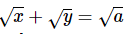at any point on it cut the axes OX and OY at P and Q respectively. Then OP + OQ equals

Solution:
QUESTION: 53

The value of tan 20º + 2 tan 50º − tan 70º is:

Solution:
QUESTION: 54

In an acute angled triangle ABC, the least value of sec A + sec B + sec C is

Solution:
QUESTION: 55

ABC is a triangle, the point P is on side BC such that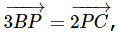, the point Q is on the line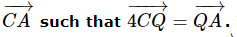. The ratio in which the line joining the common point R of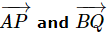and the point C divides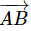is:

Solution:
QUESTION: 56

Let A = N x N, and let ' ∗ ' be a binary operation on A defined by (a,b) ∗ (c,d) = (ad+bc,bd) for all (a,b), (c,d) ∈ N x N. Then find identity element in A.

Solution:
QUESTION: 57

If the solution set for f (x) < 3 is (0 , ∞) and the solution set for f (x) > − 2 is  (−∞ , 5), then the true solution set for (f (x))2  ≥ f (x) + 6 , is

Solution:
QUESTION: 58

Let P(3, 2, 6) be a point in space and Q be a point on the line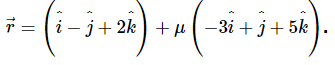Then the value of μ for which the vector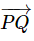is parallel to the plane x - 4y+3z = 1 is

Solution:
QUESTION: 59

If tan (π cos θ) = cot (π sin θ) , then the value(s) of cos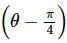is/are

Solution:
QUESTION: 60

Let f (x) = min(x, x2), for every real number x then,

Solution:
QUESTION: 61

Tangents are drawn to a unit circle with centre at (0, 0) from each point on the line 2x + y = 4, then the locus of mid-points of chord of contact is

Solution:
QUESTION: 62

Two of the lines represented by x3 − 6x2y + 3xy2 + dy3 = 0 are perpendicular for

Solution:
QUESTION: 63

There are N boxes, each containing at most r balls. If the number of boxes containing at least i balls is Ni for i = 1, 2, ....r, then the total number of balls contained in these N boxes is

Solution:
QUESTION: 64

If 0º < θ < 180º then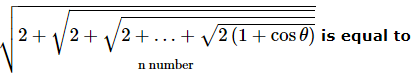Solution:
QUESTION: 65

Statement-1 :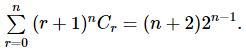Statement-2 :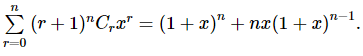Solution:
*Multiple options can be correct
QUESTION: 66

Let α ∈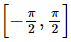and cos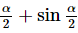=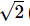(sin 54º − cos 72º) , then the value of α must be

Solution:
QUESTION: 67

Let A(z1), B(z2), C(z3) be the vertices of a triangle in the Argand plane. then which of the following statements is correct?

Solution:
*Multiple options can be correct
QUESTION: 68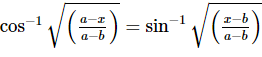is possible, if

Solution:
*Multiple options can be correct
QUESTION: 69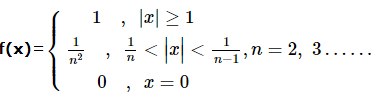Solution:
*Multiple options can be correct
QUESTION: 70

If M ans N are two events, the pobability that exactly one of them occurs is

Solution:
*Multiple options can be correct
QUESTION: 71

The equation of the line which divides the distance between the lines x - y - 7 = 0 and x - y + 3 = 0 in the ratio of 3 : 2 can be

Solution:
*Multiple options can be correct
QUESTION: 72

The equation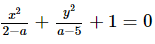represents an ellipse if a ∈

Solution:
*Multiple options can be correct
QUESTION: 73

The range of values of 'a' such that angle θ between the pair of tangents drawn from (a, 0) to the circle x2 + y2 = 1 satisfies π/2 < θ < π , lies in

Solution:
*Multiple options can be correct
QUESTION: 74

The tangents drawn from (0, 0) to x2 + y2 + 2fy + 2gx + c = 0 are perpendicular if (where c = g2)

Solution:
*Multiple options can be correct
QUESTION: 75

If 8 sin θ + 7 cos θ = 8 , then the value of 7 sin θ − 8 cos θ is equal to

Solution:Use Code STAYHOME200 and get INR 200 additional OFF Use Coupon Code